Like   Tweet   +1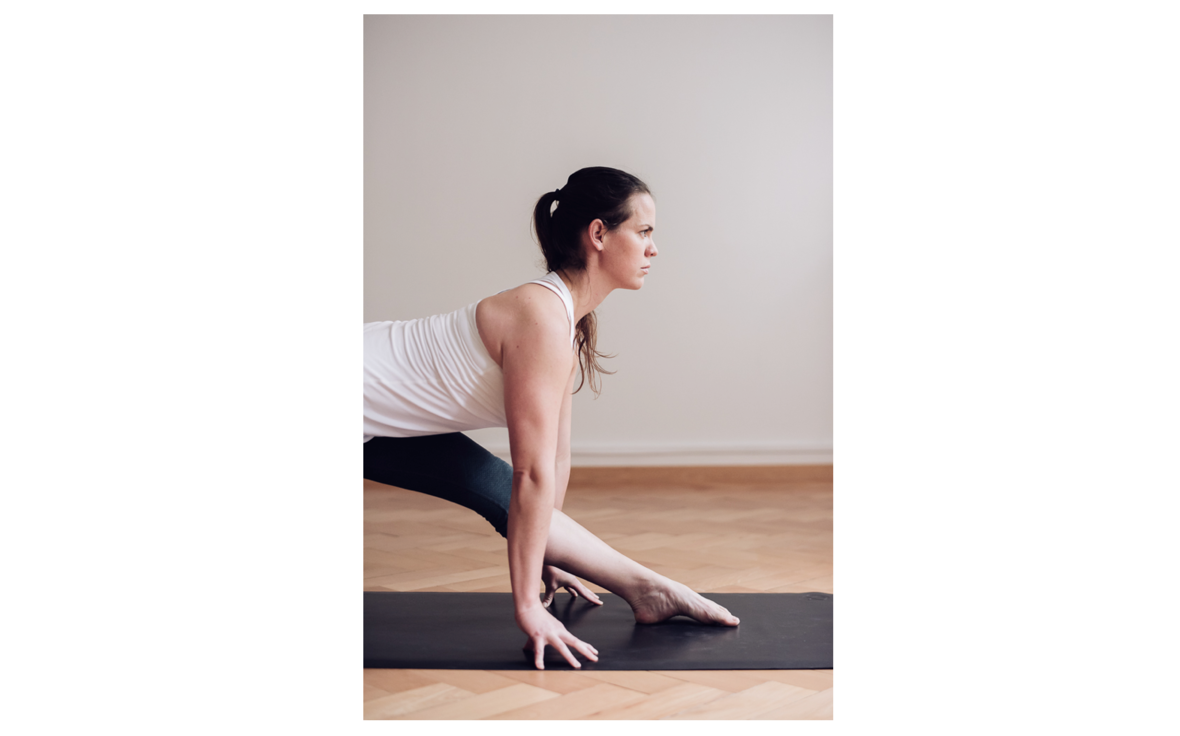## January 2019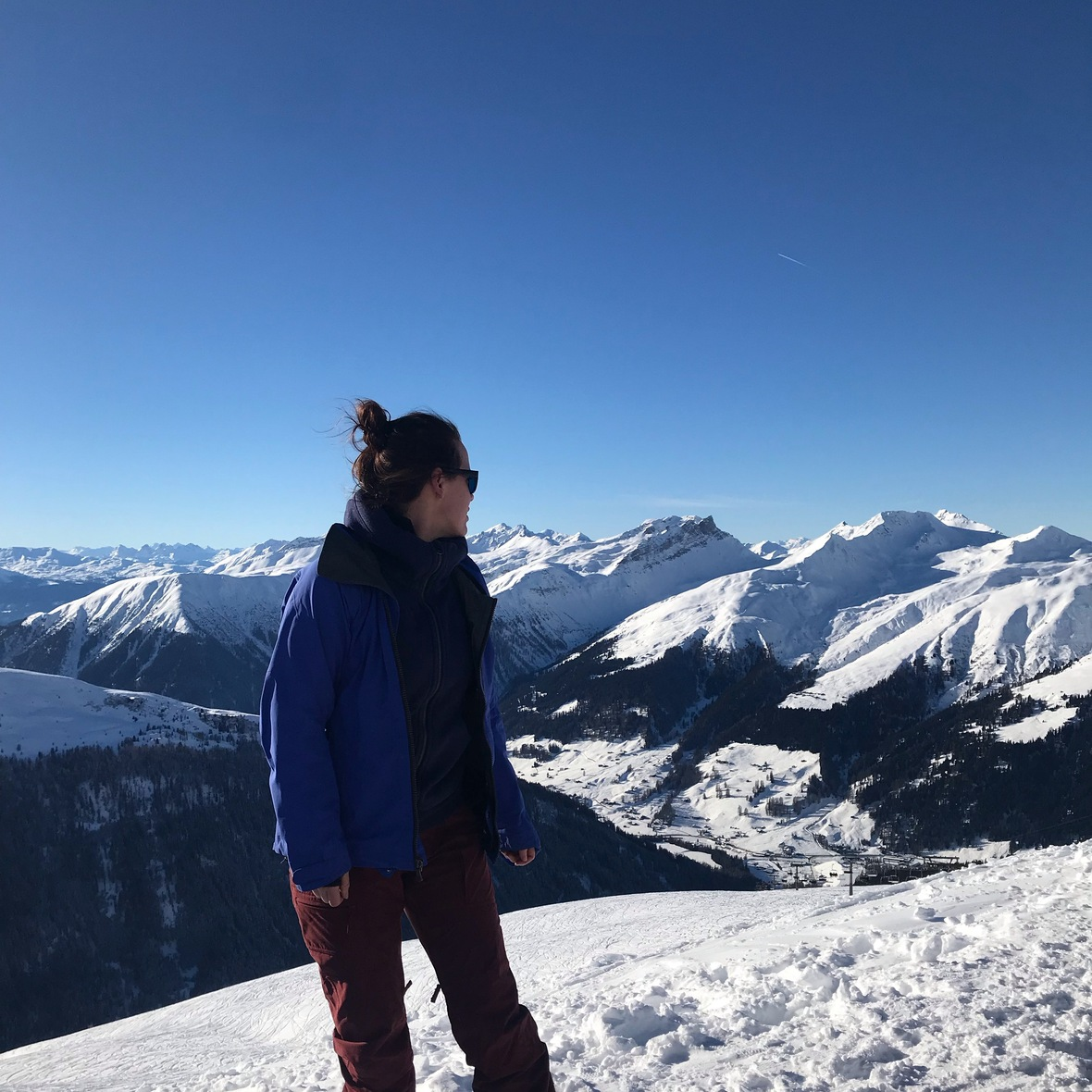First Day of 365 Happy New Year Yogi!

## Dear Yogi!

Whoa! One week into 2019! How do you feel? Yesterday's class in Thalwil was great, we had such amazing energy in the room! It was great to see some of you that I haven't seen for a while! WELCOME BACK on the mat!

Lots of YOGA things are happening in January for you! Check out the listings below and make sure to email me and reserve your mat space! (I am working on an online sign up variation... Really. It is coming! What can I say, I am an old-skool kid.)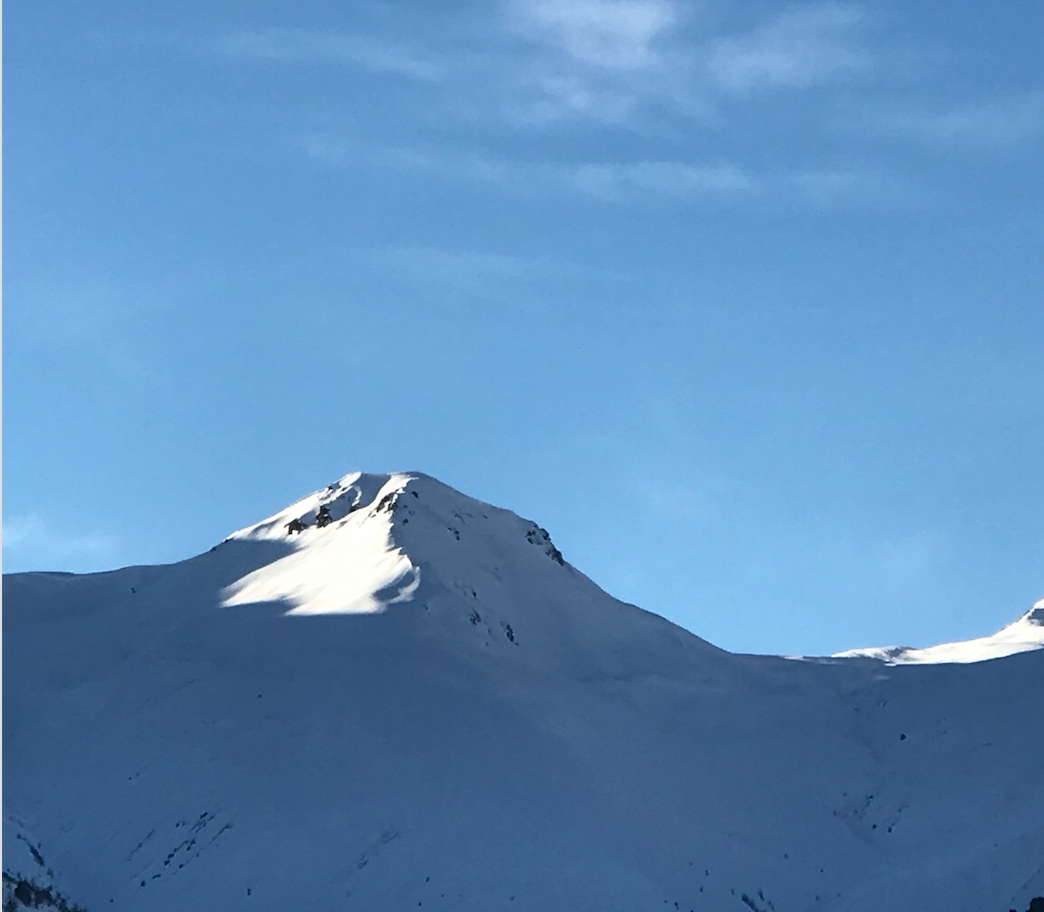Some impressions on how I started 2019!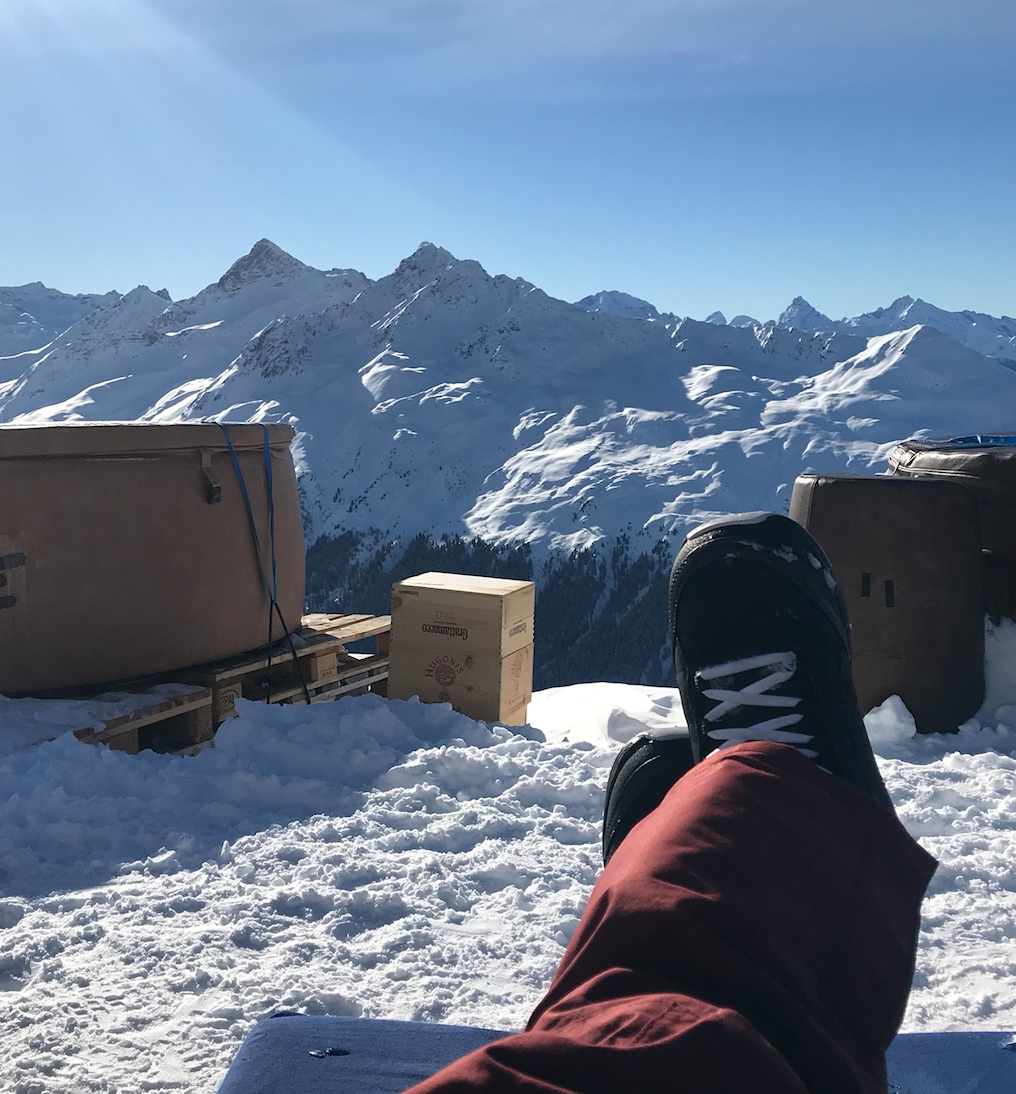table div table+table+table+table+table+table div table{width:100%;padding:0}table div table+table+table+table+table+table div table img{width:96.23%;padding:0;float:none}table div table+table+table+table+table+table div table td{width:100%;padding:0 1.88% 18px}/* styles */## Saturday Practice + Meditation

First and foremost I would love to see you this Saturday January 12
at The Space in Zurich from 10:00 - 11:45.
I am teaching Practice + Meditation and our focus will be on breathing, the spine and our gut! Any guesses to what we'll do? :-D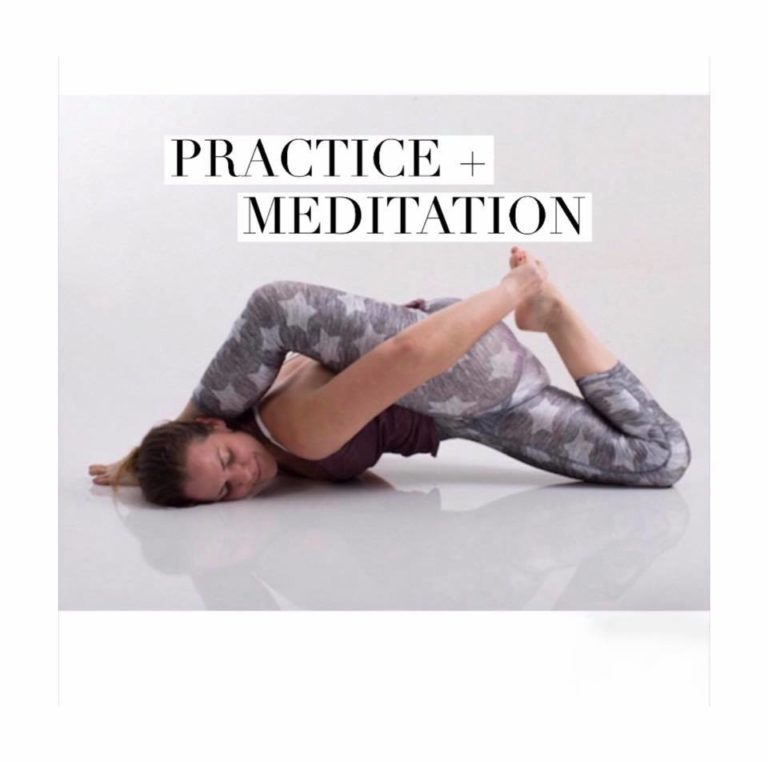This Saturday! Don't miss YOGA!

Curious to what the practice is all about?
It is deep practice and for you if you need to quiet your monkey mind! This is a Vinyasa / Flow style class that is taught with a side of magic and stardust. Built upon the foundations Hanuman Flow, it is inventive and fun and can have a little bit of everything; flow, inversions, breath-work, core, twists, and seated postures. It is a longer practice that will give us time to immerse completely into the depths of asana and sweat. Topping it all off with a sweet and surrendering meditation to restore the craziness in your head. Calm your mind, strengthen your body and become amazingly badass or deliciously sweet. The choice is yours!
(Style: Strengthening, Vinyasa + Deep Yoga)

 table div table+table+table+table+table+table+table+table+table div table{width:100%;padding:0}table div table+table+table+table+table+table+table+table+table div table img{width:96.23%;padding:0;float:none}table div table+table+table+table+table+table+table+table+table div table td{width:100%;padding:0 1.88% 18px}/* styles */## EXTRA January YOGA Love

There are two Sweat + Relax Yoga Challenges prepared for you! I have two extra classes + a Workshop scheduled at Living Yoga in Lucerne and I will be a Guest-Teacher at Yoga Elements in Feldmeilen!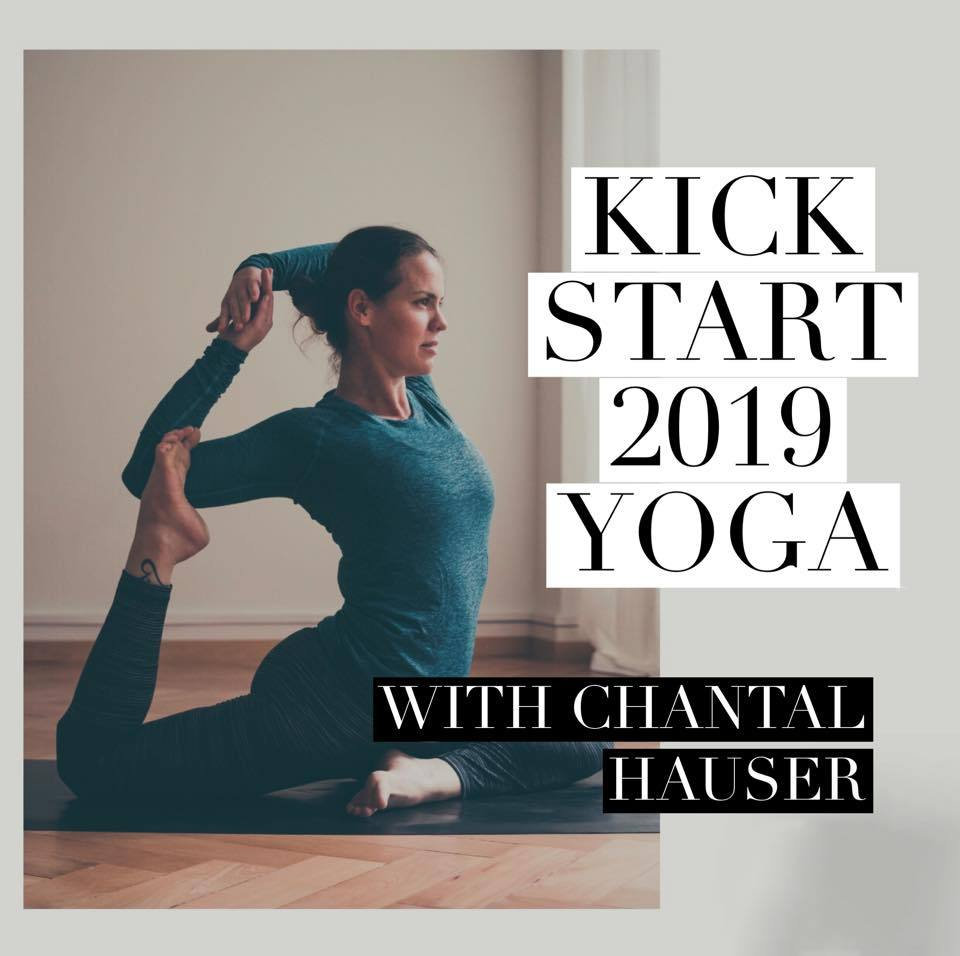MONDAYS
at lululemon Zurich
18:30 - 19:30 Sweat + Relax at lululemon, Marktgasse 12

THURSDAYS
at nyc Fit Thalwil
18:30 - 19:30 Sweat + Relax at NYC Fit, Bönirainstrasse 12

 table div table+table+table+table+table+table+table+table+table+table+table+table div table{width:100%;padding:0}table div table+table+table+table+table+table+table+table+table+table+table+table div table img{width:96.23%;padding:0;float:none}table div table+table+table+table+table+table+table+table+table+table+table+table div table td{width:100%;padding:0 1.88% 18px}/* styles */## More Happenings + FACTS!

Guest Teaching at Yoga Elements
Join me every January Tuesday from 09:00-10:30 for my Hanuman Flow at Yoga Elements, General-Wille-Str. 108, 8706 Feldmeilen.
Facebook Event | Drop In: 40.- chf or YE Abo

A Wednesday AM at Birdhaus
I am teaching a FREE for Members yoga hour at Birdhaus this month! Get some productive work done after a light and great start into Wednesday, January 16 from 9:00-10:00 at Birdhaus, Brandschenkestrasse 150, 8002 Zürich.

Living Yoga on Sundays
Come and join me for my two last Sundays at Living Yoga in Lucerne! I am teaching on January 13 and February 10 from 10:00-11:15.

Highway to Hamstrings Workshop
Do you want to feel your legs in a really interesting way? Especially after half of skiing season is over? Then this workshop I am having at Living Yoga is for you! Practice with me on Saturday, January 26 from 11:00-13:00!
Find the full description HERE and use your legs to fly! :-D
Facebook Event | Monetas: 60.- chf

Special Price | Jackie D. is teaching for me!
I am going out (Orchestra Night) on Sunday, January 27 and Jackie will teach a Yoga Flow from 16:30 - 18:00 at Malayoga, Gotthardstrasse 32 in Thalwil!
Drop In: 35.- chf | Please register chantalhauseryoga@gmail.com

Open Private Sessions for Friday
From January 25 to February 8 I have a private slot left from 13:30 - 14:30 in Thalwil! It can also be booked as a Group Private! Email, text or call me to book it!

Mom + Babe Course on Mondays
Our adorable and fierce Monday morning with Mom + Babe Yoga is continuing. There is one spot left for January 14 | 21 and 28! We meet at Malayoga, Gotthardstrasse 32 in Thalwil from 11:15-12:15 and there is enough space to change, to feed and to yoga with your baby!
Invest: 150.- chf for the full package | Facebook Event

 table div table+table+table+table+table+table+table+table+table+table+table+table+table+table div table{width:100%;padding:0}table div table+table+table+table+table+table+table+table+table+table+table+table+table+table div table img{width:96.23%;padding:0;float:none}table div table+table+table+table+table+table+table+table+table+table+table+table+table+table div table td{width:100%;padding:0 1.88% 18px}/* styles */table div table+table+table+table+table+table+table+table+table+table+table+table+table+table+table div table{width:100%;padding:0}table div table+table+table+table+table+table+table+table+table+table+table+table+table+table+table div table img{width:96.23%;padding:0;float:none}table div table+table+table+table+table+table+table+table+table+table+table+table+table+table+table div table td{width:100%;padding:0 1.88% 18px}/* styles *//* styles */ I have got some more writing done over the Holidays. It has been a great time of solitude for me. The snow has added to my clarity and I am looking forward to what 2019 has in store for me and for us! Enjoy your first week back on track! Or if you are not quite there yet, it is okay! Come to yoga in the meantime! Much Love + Peace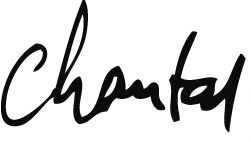## P.S.

Enjoy the freshness of the year! I wish you a healthy and happy 2019 Keep practicing and breathing as always!

 table div table+table+table+table+table+table+table+table+table+table+table+table+table+table+table+table+table+table+table div table{width:100%;padding:0}table div table+table+table+table+table+table+table+table+table+table+table+table+table+table+table+table+table+table+table div table img{width:96.23%;padding:0;float:none}table div table+table+table+table+table+table+table+table+table+table+table+table+table+table+table+table+table+table+table div table td{width:100%;padding:0 1.88% 18px}/* styles */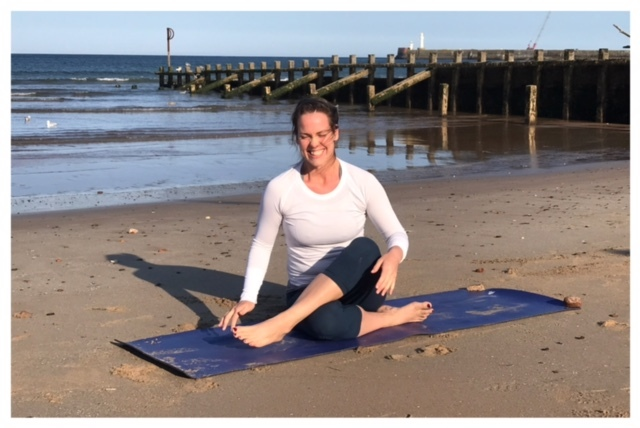table div table+table+table+table+table+table+table+table+table+table+table+table+table+table+table+table+table+table+table+table+table+table div table{width:100%;padding:0}table div table+table+table+table+table+table+table+table+table+table+table+table+table+table+table+table+table+table+table+table+table+table div table img{width:96.23%;padding:0;float:none}table div table+table+table+table+table+table+table+table+table+table+table+table+table+table+table+table+table+table+table+table+table+table div table td{width:100%;padding:0 1.88% 18px}/* styles */## Tuesday - Dienstag

12:15 - 13:15 Hanuman Flow (EN)
at The Space in Zürich | K5

17:30 - 18:45 Basic + Neu (CH)
at Living Yoga in Luzern

19:00 - 20:30 Hanuman Flow (EN)
at Living Yoga in Luzern

 table div table+table+table+table+table+table+table+table+table+table+table+table+table+table+table+table+table+table+table+table+table+table+table+table div table{width:100%;padding:0}table div table+table+table+table+table+table+table+table+table+table+table+table+table+table+table+table+table+table+table+table+table+table+table+table div table img{width:96.23%;padding:0;float:none}table div table+table+table+table+table+table+table+table+table+table+table+table+table+table+table+table+table+table+table+table+table+table+table+table div table td{width:100%;padding:0 1.88% 18px}/* styles */## Saturday - Samstag

This class takes place 1x a month - every second Saturday
10:00 - 11:45 Practice + Meditation (EN)
at The Space in Zürich | K5

 table div table+table+table+table+table+table+table+table+table+table+table+table+table+table+table+table+table+table+table+table+table+table+table+table+table+table div table{width:100%;padding:0}table div table+table+table+table+table+table+table+table+table+table+table+table+table+table+table+table+table+table+table+table+table+table+table+table+table+table div table img{width:96.23%;padding:0;float:none}table div table+table+table+table+table+table+table+table+table+table+table+table+table+table+table+table+table+table+table+table+table+table+table+table+table+table div table td{width:100%;padding:0 1.88% 18px}/* styles */## Sunday - Sonntag

16:30 - 18:00 Hanuman Flow (EN)
at Malayoga in Thalwil

 table div table+table+table+table+table+table+table+table+table+table+table+table+table+table+table+table+table+table+table+table+table+table+table+table+table+table+table+table div table{width:100%;padding:0}table div table+table+table+table+table+table+table+table+table+table+table+table+table+table+table+table+table+table+table+table+table+table+table+table+table+table+table+table div table img{width:96.23%;padding:0;float:none}table div table+table+table+table+table+table+table+table+table+table+table+table+table+table+table+table+table+table+table+table+table+table+table+table+table+table+table+table div table td{width:100%;padding:0 1.88% 18px}/* styles */# Kids Yoga Classes

## Thursdays - Donnerstags

16:00 - 17:00 Yoga Club (EN)
at Lakeside School in Horgen

## Fridays - Freitags

09:30 - 11:00 Kids Yoga (EN)
at Kids Residence in Rüschlikon

 table div table+table+table+table+table+table+table+table+table+table+table+table+table+table+table+table+table+table+table+table+table+table+table+table+table+table+table+table+table+table div table{width:100%;padding:0}table div table+table+table+table+table+table+table+table+table+table+table+table+table+table+table+table+table+table+table+table+table+table+table+table+table+table+table+table+table+table div table img{width:96.23%;padding:0;float:none}table div table+table+table+table+table+table+table+table+table+table+table+table+table+table+table+table+table+table+table+table+table+table+table+table+table+table+table+table+table+table div table td{width:100%;padding:0 1.88% 18px}/* styles */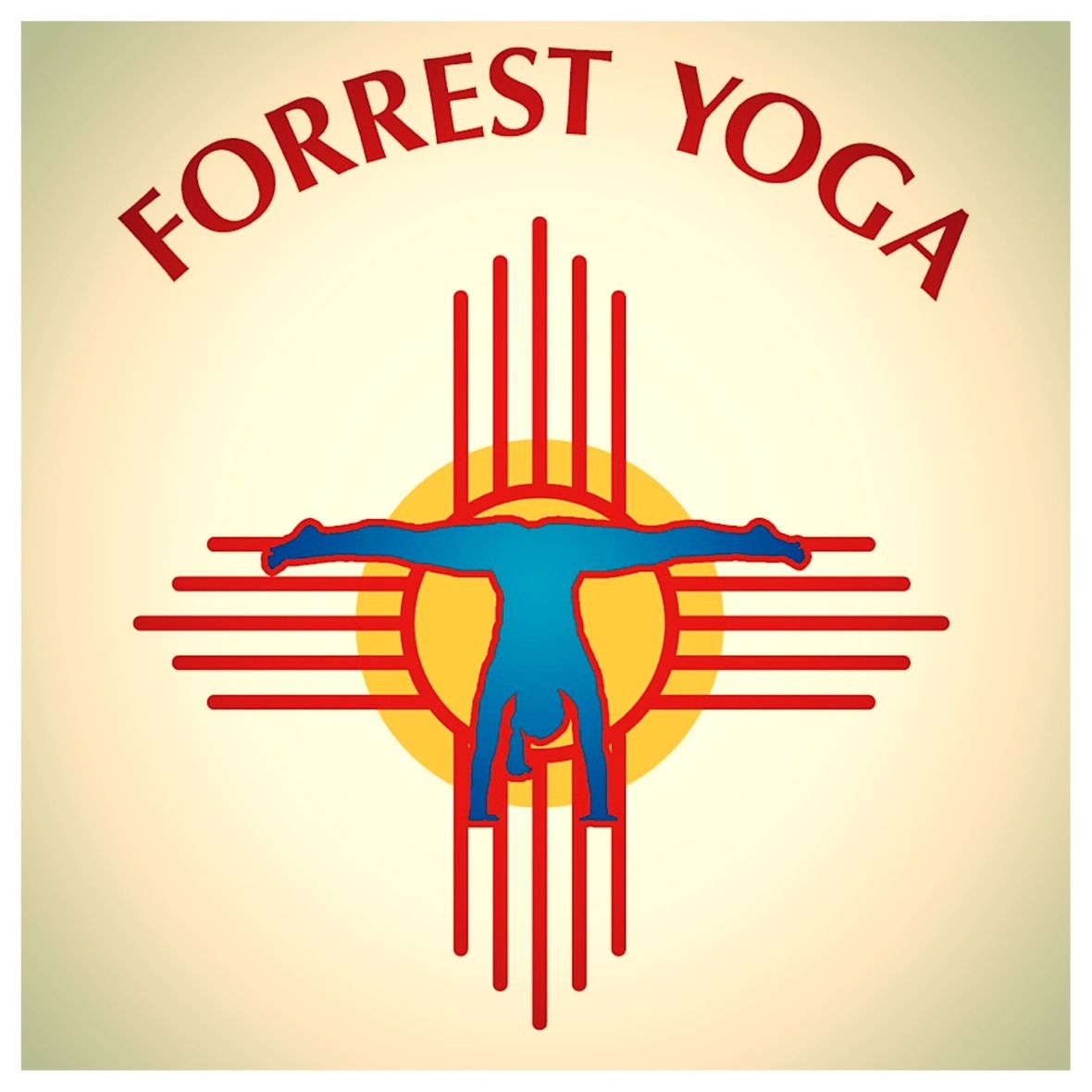## Forrest Yoga Intensive

Friday | February 1
18:30 - 20:30 Forrest Yoga Intensive (E)
Perfectionism | Roll Work + Core Connection

We will deepen our body love in this Friday Intensive by looking at our perfectionism and the fear of missing out. The sequence is built around mini backbends and twists. Get up close with the Forrest Yoga roll and get connected to your gut and the feelings that we harvest in that area.

at Body Love Fitness in Zürich | Seefeld
Invest: 75.- chf | Sign Me Up | Facebook Event

 table div table+table+table+table+table+table+table+table+table+table+table+table+table+table+table+table+table+table+table+table+table+table+table+table+table+table+table+table+table+table+table+table+table div table{width:100%;padding:0}table div table+table+table+table+table+table+table+table+table+table+table+table+table+table+table+table+table+table+table+table+table+table+table+table+table+table+table+table+table+table+table+table+table div table img{width:96.23%;padding:0;float:none}table div table+table+table+table+table+table+table+table+table+table+table+table+table+table+table+table+table+table+table+table+table+table+table+table+table+table+table+table+table+table+table+table+table div table td{width:100%;padding:0 1.88% 18px}/* styles */## Saturday Practice + Meditation

Saturday | February 9
10:00 - 11:45 Practice + Meditation (E)

at The Space in Zürich | K5
Invest: 45.- chf | Sign Me Up

 table div table+table+table+table+table+table+table+table+table+table+table+table+table+table+table+table+table+table+table+table+table+table+table+table+table+table+table+table+table+table+table+table+table+table+table div table{width:100%;padding:0}table div table+table+table+table+table+table+table+table+table+table+table+table+table+table+table+table+table+table+table+table+table+table+table+table+table+table+table+table+table+table+table+table+table+table+table div table img{width:96.23%;padding:0;float:none}table div table+table+table+table+table+table+table+table+table+table+table+table+table+table+table+table+table+table+table+table+table+table+table+table+table+table+table+table+table+table+table+table+table+table+table div table td{width:100%;padding:0 1.88% 18px}/* styles */## Deep Immersion Training - 15hrs

Friday-Sunday | March 22-24
Starts 18:30 - Ends 15:00
Weekend Immersion
(E)

This is for you if you can answer at least one with YES;

▪ You have been a long time Yogi, haven’t done a Teacher Training yet but maybe want to soon. OR you just want to practice!
▪ You are a Yoga Teacher and have done the 200 / 300 etc. and gazillion of other Yoga Teacher Trainings and are on the look out for THE next training that will really up level your practice.
▪ You are looking for a personalized and intimate setting with an absolute max. of 10 other Yogis in the group.
▪ You want to immerse yourself in a weekend of intelligent sequencing, assists, refresh on yoga philosophy, deepen your knowledge on Pranayama and the Bandhas.
▪ You want to RETREAT and have weekend to yourself on the yoga mat but not travel around the world to get there!
 ▪ You have been a long time Yogi, haven’t done a Teacher Training yet but maybe want to soon. OR you just want to practice!
 ▪ You are a Yoga Teacher and have done the 200 / 300 etc. and gazillion of other Yoga Teacher Trainings and are on the look out for THE next training that will really up level your practice.
 ▪ You are looking for a personalized and intimate setting with an absolute max. of 10 other Yogis in the group.
 ▪ You want to immerse yourself in a weekend of intelligent sequencing, assists, refresh on yoga philosophy, deepen your knowledge on Pranayama and the Bandhas.
 ▪ You want to RETREAT and have weekend to yourself on the yoga mat but not travel around the world to get there!

at The Space in Zürich | K5
Early Bird: 495.- chf (EXTENDED to January 11, 2019 because I know time flies way too fast!)
Investment: 600.- chf (paid by March 1, 2019)

 table div table+table+table+table+table+table+table+table+table+table+table+table+table+table+table+table+table+table+table+table+table+table+table+table+table+table+table+table+table+table+table+table+table+table+table+table+table div table{width:100%;padding:0}table div table+table+table+table+table+table+table+table+table+table+table+table+table+table+table+table+table+table+table+table+table+table+table+table+table+table+table+table+table+table+table+table+table+table+table+table+table div table img{width:96.23%;padding:0;float:none}table div table+table+table+table+table+table+table+table+table+table+table+table+table+table+table+table+table+table+table+table+table+table+table+table+table+table+table+table+table+table+table+table+table+table+table+table+table div table td{width:100%;padding:0 1.88% 18px}/* styles */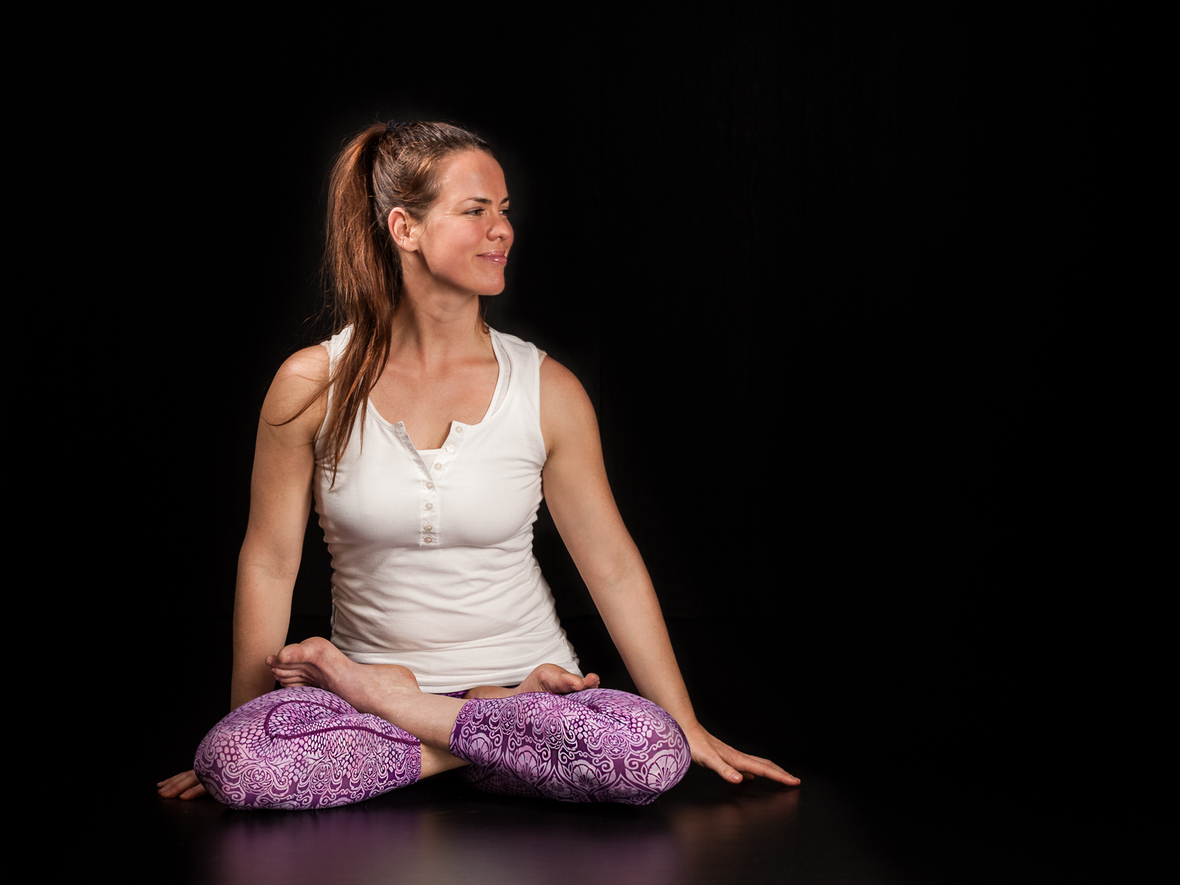## Writing on YogaWiser

I was able to get inspired and write a lot during the holidays! Mostly because I did allow myself space to breathe and be.

Take a couple of minutes to read my newest article What is to Love in this Lifetime? by clicking HERE and if you like poems read Snow - A Poem by clicking here!

I am always open to know what you think about it or if it made you think about something! Feel free to email me and share your thoughts!

 table div table+table+table+table+table+table+table+table+table+table+table+table+table+table+table+table+table+table+table+table+table+table+table+table+table+table+table+table+table+table+table+table+table+table+table+table+table+table+table+table div table{width:100%;padding:0}table div table+table+table+table+table+table+table+table+table+table+table+table+table+table+table+table+table+table+table+table+table+table+table+table+table+table+table+table+table+table+table+table+table+table+table+table+table+table+table+table div table img{width:96.23%;padding:0;float:none}table div table+table+table+table+table+table+table+table+table+table+table+table+table+table+table+table+table+table+table+table+table+table+table+table+table+table+table+table+table+table+table+table+table+table+table+table+table+table+table+table div table td{width:100%;padding:0 1.88% 18px}/* styles */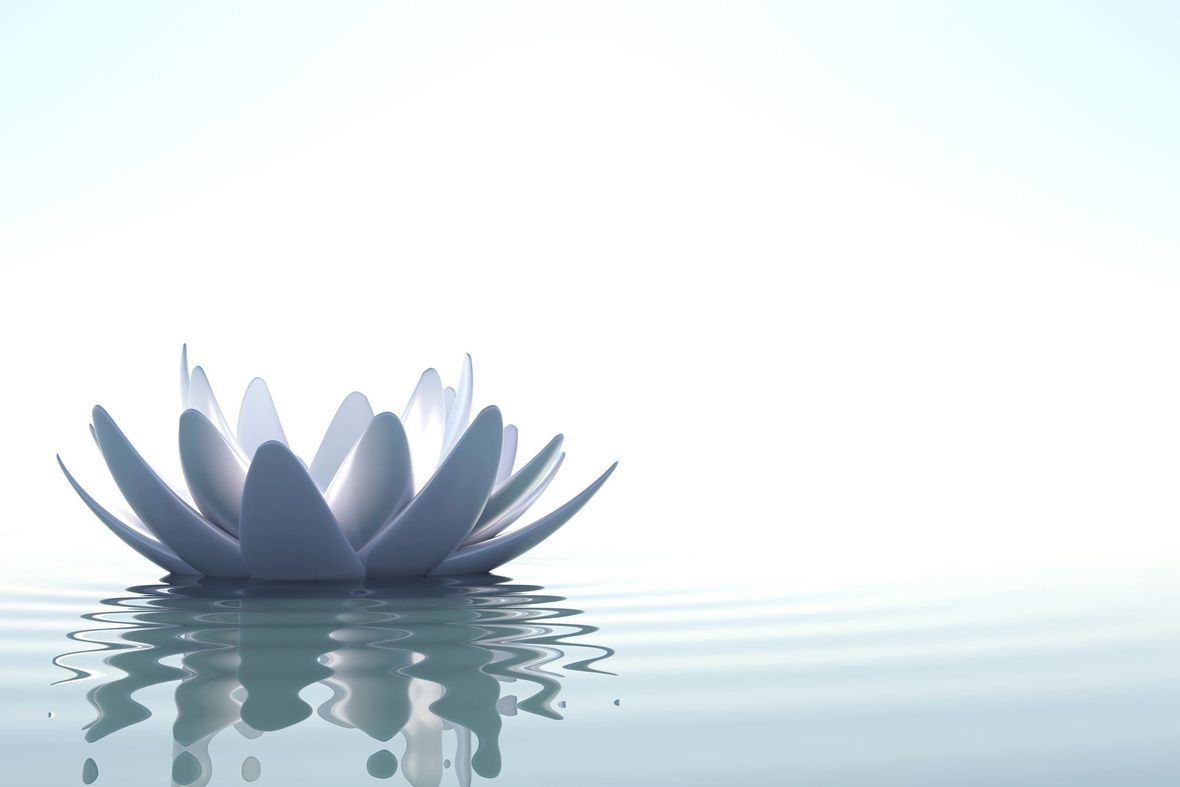Start Your Tree Map in January!

## Intention for January

Allowing Space
January is usually a big "resolution, planning to get in shape kind of time". However, allow yourself space to grow and figure out what your plans are for the rest of the year. Know that they will change as well.

Personally, I like to draw and color and start a My Tree Map. I suppose it is similar to a vision board. Mine usually ends up totally chaotic (because that is life!) by the end of the year, but I do use it to look back at my year.

In case you do not know, I am a big fan of lists! My Tree Map starts out like a tree where I list my foundations, my ethics, people I love and 3 things that make me happy. Then I draw little roads around the tree (kind of like a Monopoly road) and write down the places I want to go and see, I pick 3 things I want to change in my life (for example; having a screen-less day during the week), etc. I write down "goals" and "dreams" as well as "hopes". I use it throughout the year to check in with my little roads around the tree and also know that they can change. I have been doing it for 4 years now and it is really cool to track and see your changes!

What do you say? Are you ready to draw a litte Tree Map of your own?
All you need is a piece of paper and your favourite pen!

 table div table+table+table+table+table+table+table+table+table+table+table+table+table+table+table+table+table+table+table+table+table+table+table+table+table+table+table+table+table+table+table+table+table+table+table+table+table+table+table+table+table+table div table{width:100%;padding:0}table div table+table+table+table+table+table+table+table+table+table+table+table+table+table+table+table+table+table+table+table+table+table+table+table+table+table+table+table+table+table+table+table+table+table+table+table+table+table+table+table+table+table div table img{width:96.23%;padding:0;float:none}table div table+table+table+table+table+table+table+table+table+table+table+table+table+table+table+table+table+table+table+table+table+table+table+table+table+table+table+table+table+table+table+table+table+table+table+table+table+table+table+table+table+table div table td{width:100%;padding:0 1.88% 18px}/* styles */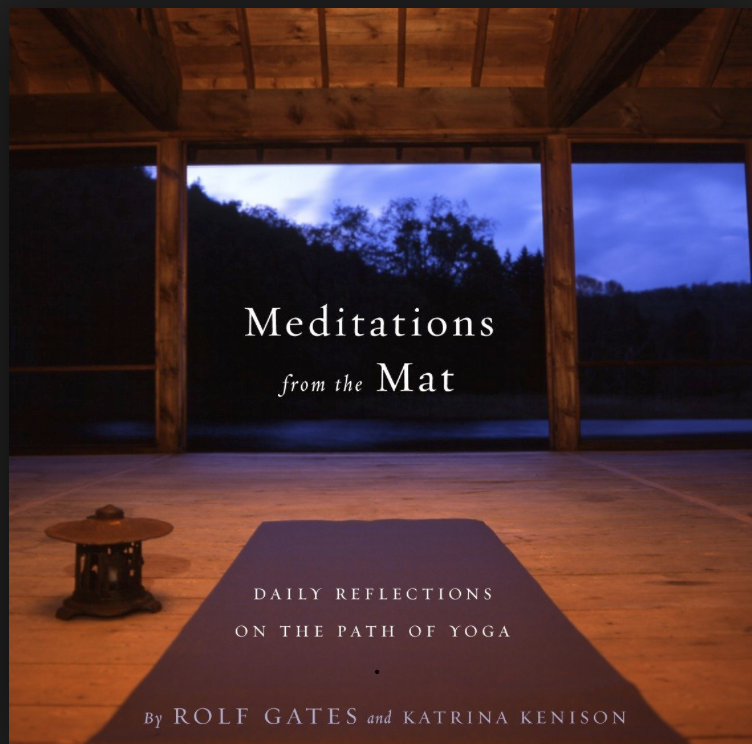## Book Corner

A good friend of mine gifted me this book a couple of year back. I just left university and a good chunk of my life behind! Meditations from the Mat by Rolf Gates and Katrina Kenison has helped me so much in a very subtle way. I am usually not the quotation a day kind of gal but I highly recommend this book if you are going through a transition.

 table div table+table+table+table+table+table+table+table+table+table+table+table+table+table+table+table+table+table+table+table+table+table+table+table+table+table+table+table+table+table+table+table+table+table+table+table+table+table+table+table+table+table+table+table div table{width:100%;padding:0}table div table+table+table+table+table+table+table+table+table+table+table+table+table+table+table+table+table+table+table+table+table+table+table+table+table+table+table+table+table+table+table+table+table+table+table+table+table+table+table+table+table+table+table+table div table img{width:96.23%;padding:0;float:none}table div table+table+table+table+table+table+table+table+table+table+table+table+table+table+table+table+table+table+table+table+table+table+table+table+table+table+table+table+table+table+table+table+table+table+table+table+table+table+table+table+table+table+table+table div table td{width:100%;padding:0 1.88% 18px}/* styles */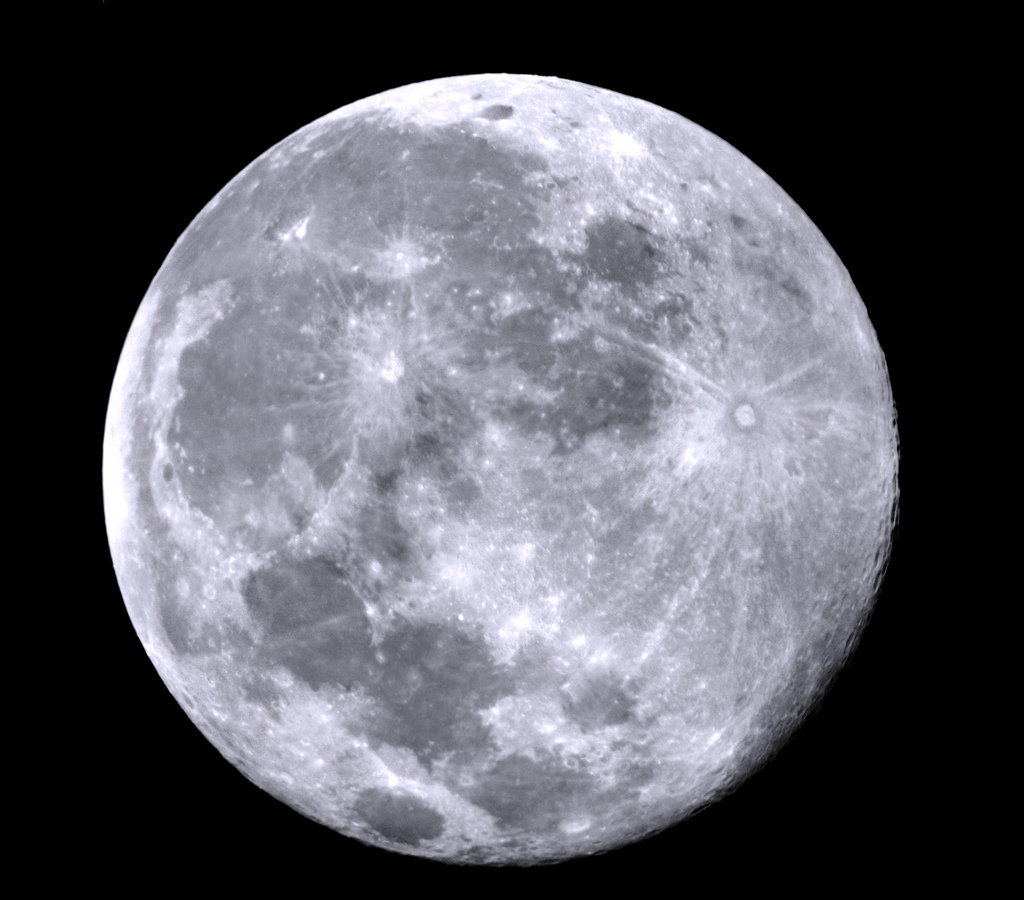An important reminder of change!

## Moon Days January

New Moon
Sunday 6.1 | 02:28
It was yesterday, think back, did you feel a bit more grounded?

Full Moon / Super + Wolf Moon
Monday 26.12 | 06:16
There is also a Lunar Eclipse that morning!

 table div table+table+table+table+table+table+table+table+table+table+table+table+table+table+table+table+table+table+table+table+table+table+table+table+table+table+table+table+table+table+table+table+table+table+table+table+table+table+table+table+table+table+table+table+table+table div table{width:100%;padding:0}table div table+table+table+table+table+table+table+table+table+table+table+table+table+table+table+table+table+table+table+table+table+table+table+table+table+table+table+table+table+table+table+table+table+table+table+table+table+table+table+table+table+table+table+table+table+table div table img{width:96.23%;padding:0;float:none}table div table+table+table+table+table+table+table+table+table+table+table+table+table+table+table+table+table+table+table+table+table+table+table+table+table+table+table+table+table+table+table+table+table+table+table+table+table+table+table+table+table+table+table+table+table+table div table td{width:100%;padding:0 1.88% 18px}/* styles */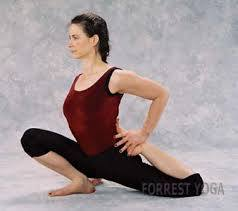## Asana Mash-Up

This pose is from Forrest Yoga and is called Lunge H-B. The H-B means Heel to Butt as you can see in the picture of Ana. She is very deep forward in her lunging, your B might not come nearly as close to the floor as hers, but this is a fave posture during Winter Season, especially if you have tight quads and shins! Use a blanket under your knees and do not push on bone. Use a block and a strap like we usually do during our classes. Watch out for this posture, it will show up during our January classes!

 table div table+table+table+table+table+table+table+table+table+table+table+table+table+table+table+table+table+table+table+table+table+table+table+table+table+table+table+table+table+table+table+table+table+table+table+table+table+table+table+table+table+table+table+table+table+table+table+table div table{width:100%;padding:0}table div table+table+table+table+table+table+table+table+table+table+table+table+table+table+table+table+table+table+table+table+table+table+table+table+table+table+table+table+table+table+table+table+table+table+table+table+table+table+table+table+table+table+table+table+table+table+table+table div table img{width:96.23%;padding:0;float:none}table div table+table+table+table+table+table+table+table+table+table+table+table+table+table+table+table+table+table+table+table+table+table+table+table+table+table+table+table+table+table+table+table+table+table+table+table+table+table+table+table+table+table+table+table+table+table+table+table div table td{width:100%;padding:0 1.88% 18px}/* styles */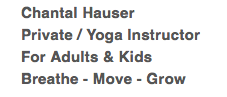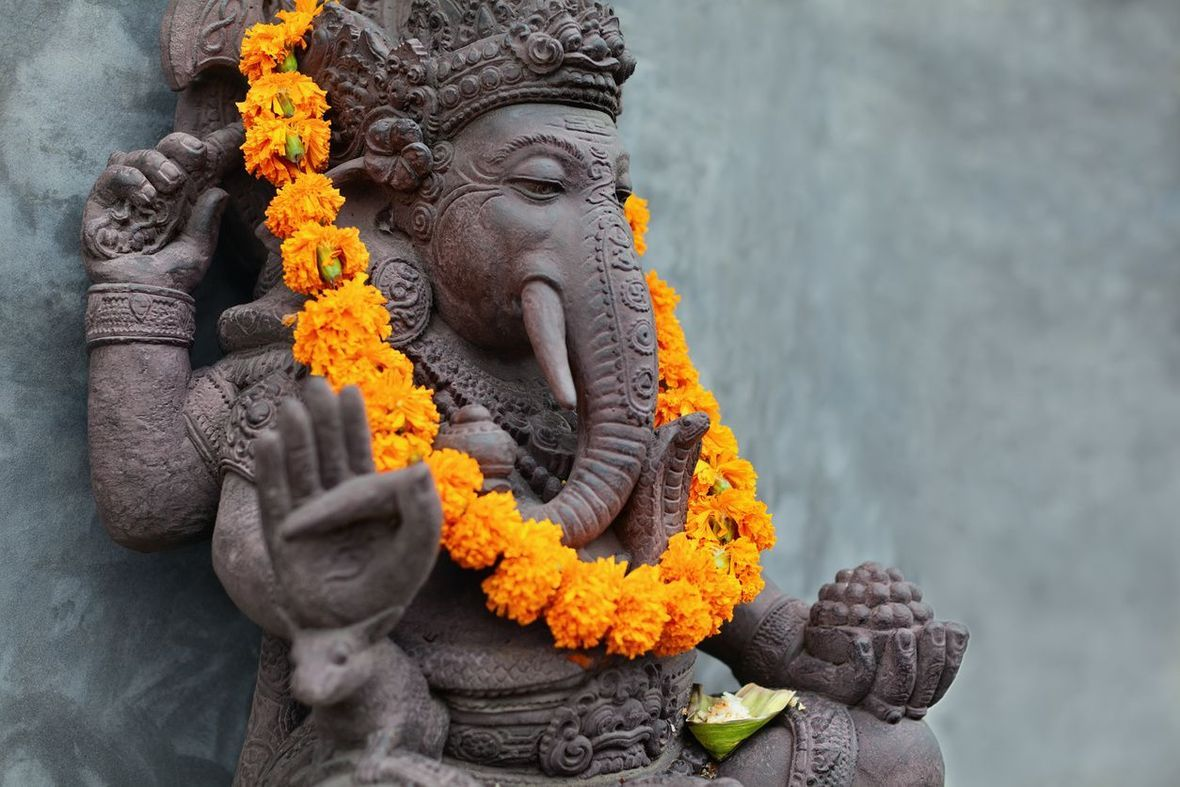table div table+table+table+table+table+table+table+table+table+table+table+table+table+table+table+table+table+table+table+table+table+table+table+table+table+table+table+table+table+table+table+table+table+table+table+table+table+table+table+table+table+table+table+table+table+table+table+table+table+table+table+table div table{width:100%;padding:0}table div table+table+table+table+table+table+table+table+table+table+table+table+table+table+table+table+table+table+table+table+table+table+table+table+table+table+table+table+table+table+table+table+table+table+table+table+table+table+table+table+table+table+table+table+table+table+table+table+table+table+table+table div table img{width:96.23%;padding:0;float:none}table div table+table+table+table+table+table+table+table+table+table+table+table+table+table+table+table+table+table+table+table+table+table+table+table+table+table+table+table+table+table+table+table+table+table+table+table+table+table+table+table+table+table+table+table+table+table+table+table+table+table+table+table div table td{width:100%;padding:0 1.88% 18px}/* styles */